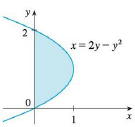Chapter 5.4, Problem 49E

Chapter
Section
Textbook Problem

The area of the region that lies to the right of the y-axis and to the left of the parabola x = 2y − y2 (the shaded region in the figure) is given by the integral ∫ 0 2 ( 2 y − y 2 )   d y . (Turn your head clockwise and think of the region as lying below the curve x = 2y − y2 from y = 0 to y = 2.) Find the area of the region.To determine

To find: The area of the shaded region.

Explanation

Given information:

The shaded region is bounded by a curve with x=2yy2.

The region lies between y=0 and y=2.

Find the area of the shaded region (A) as shown below:

A=abxdy (1)

Here, the curve function is x, the lower limit is a, and the upper limit is b.

Substitute 2yy2 for x, 2 for b, and 0 for a in Equation (1).

A=02(2yy2)dy=[2

Still sussing out bartleby?

Check out a sample textbook solution.

See a sample solution

The Solution to Your Study Problems

Bartleby provides explanations to thousands of textbook problems written by our experts, many with advanced degrees!

Get Started

Find the differential of each function. 12. (a) y=1+2u1+3u (b) y = 2 sin 2

Single Variable Calculus: Early Transcendentals, Volume I

In Problems 15-28, find the general solution to the given differential equation.

Mathematical Applications for the Management, Life, and Social Sciences

For f(x)=exx,f(x)= a) ex b) xex1x2 c) exxexx2 d) xexxx2

Study Guide for Stewart's Single Variable Calculus: Early Transcendentals, 8th